×#### Thank you for registering.

One of our academic counsellors will contact you within 1 working day.

Click to Chat

1800-1023-196

+91-120-4616500

CART 0

• 0

MY CART (5)

Use Coupon: CART20 and get 20% off on all online Study Material

ITEM
DETAILS
MRP
DISCOUNT
FINAL PRICE
Total Price: Rs.

There are no items in this cart.
Continue Shopping```Solved ExamplesExample 1:

Find the coefficient of the independent term of x in expansion of  (3x - (2/x2))15.

Solution:

The general term of (3x - (2/x2))15 is written, as Tr+1 = 15Cr (3x)15-r (-2/x2)r. It is independent of x if,

15 - r - 2r = 0 => r = 5

.·.   T6 = 15C5(3)10(-2)5 = - 16C5 310 25.

Example 2:

If the coefficient of (2r + 4)th and (r - 2)th terms in the expansion of (1+x)18 are equal then find the value of r.

Solution:

The general term of (1 + x)n is Tr+1 = Crxr

Hence coefficient of (2r + 4)th term will be

T2r+4 = T2r+3+1 = 18C2r+3

and coefficient or (r - 2)th term will be

Tr-2 = Tr-3+1 = 18Cr-3.

=> 18C2r+3 = 18Cr-3.

=> (2r + 3) + (r-3) = 18 (·.· nCr = nCK => r = k or r + k = n)

.·.     r = 6

Example 3:

If a1, a2, a3 and a4 are the coefficients of any four consecutive terms in the  expansion of (1+x)n then prove that:

a1/(a1+a2) + a2/(a3+a4) = 2a2/(a2+a3)

Solution:

As a1, a2, a3 and a4 are coefficients of consecutive terms, then

Let    a1 = nCr

a2 = nCr+1

a3 = nCr+2 and

a4 = nCr+3

Now  a1/(a1+a2) = nCr/(nCr+nCr+1)  = 1/(1+((n-r)/(r+1))) = (r+1)/(n+1)

Similarly, a2/(a2+a3) = (r+3)/(n+1)

Now  a3/(a3+a4) + a1/(a1+a2) = (2r+4)/(n+1)

= 2(r+1)/(n+1) = 2a2/(a2+a3)                              (Hence, proved)

Example 4:

Find out which one is larger 9950 + 10050 or 10150.

Solution:

Let's try to find out 10150 - 9950 in terms of remaining term i.e.

10150 - 9950 = (100+1)50 - (100 - 1)50

= (C0.10050 + C110049 + C2.10048 +......)

= (C010050 - C110049 + C210048 -......)

= 2[C1.10049 + C310047 +.........]

= 2[50.10049 + C310047 +.........]

= 10050 + 2[C310047 +............]

> 10050

=> 10150 > 9950 + 10050

Example 5:

Find the value of the greatest term in the expansion of √3(1+(1/√3))20.

Solution:

Let Tr+1 be the greatest term, then Tr < Tr+1 > Tr+2

Consider : Tr+1 > Tr

=> 20Cr   (1/√3)r > 20Cr-1(1/√3)r-1

=> ((20)!/(20-r)!r!) (1/(√3)r)  >  ((20)!/(21-r)!(r-1)!) (1/(√3)r-1)

=> r < 21/(√3+1)

=> r < 7.686                                               ......... (i)

Similarly, considering Tr+1 > Tr+2

=> r > 6.69                                              .......... (ii)

From (i) and (ii), we get

r = 7

Hence greatest term = T8 = 25840/9

Example 6:

Find the coefficient of x50 in the expansion of (1+x)1000 + 2x(1+x)999 + 3x2 (1+x)998 +...+ 1001x1000.

Solution:

Let S = (1 + x)1000 + 2x(1+x)999 +...+ 1000x999 (1+x) + 1001 x1000

This is an Arithmetic Geometric Series with r = x/(1+x) and d = 1.

Now  (x/(1+x)) S = x(1 + x)999 + 2x2 (1 + x)998 +...+ 1000x1000 + 1000x1001/(1+x)

Subtracting we get,

(1 - (x/(x+1))) S =(1+x)1000 + x(1+x)999 +...+ x1000 - 1001x1000/(1+x)

or S = (1+x)1001 + x(1+x)1000 + x2(1+x)999 +...+ x1000 (1+x)-1001x1001

This is G.P. and sum is

S = (1+x)1002 - x1002 - 1002x1001

So the coeff. of x50 is = 1002C50

Example 7:

Show thatnCk (sin kx) cos (n-k)x = 2n-1 sin(nx)

Solution:

We havenCk sin kx cos (n-k)x

=1/2nCk [sin (k x + nx - kx) + sin (kx - nx + kx)]

=1/2nCk sin n x + 1/2nCk sin (2kx - nx)

= 1/2 sin n xnCk 1/2 [nC0 sin (-nx) + nC1 sin (2-n)x +...

...+ nCn-1 sin (n-2)x + nCn sin nx]

= 2n-1 sin nx + 0 (as terms in bracket, which are equidistant, from end and beginning will cancel each other).

(Hence, proved)

Example 8:

If (15+6√6)2n+1 = P, then prove that P(1 - F) = 92n+1 (where F is the fractional part of P).

Solution:

We can write

P = (15+6√6)2n+1  = I + F (Where I is integral and F is the fractional part of P)

Let F' = (15+6√6)2n+1

Note:      6√6  = 14.69

=> 0 < 156√6  < 1

=> 0 < (15+6√6)2n+1  < 1

=> 0 < F' < 1

Now,  I + F = C0 (15)2n+1 + C1(15)2n 6√6  + C2 (15)2n (6√6 )2 +...

F' = C0 (15)2n+1 - C1(15)2n 6√6  + C2(15)2n-1 (6√6 )2 +...

I + F + F' = 2[C0 (15)2n+1 + C2 (15)2n-1 (6√6 )2 +...]

Term on R.H.S. is an even integer.

=>     I + F + F' = Even integer

=>     F + F' = Integer

But,   0 < F < 1 and             (F is fraction part)

0 < F' < 1

=>    0 < F + F' < 2

Hence F + F' = 1

F' = (1-f)

.·.     P(1-F) = (15 + 6√6 )2n+1 (15-6√6 )2n+1

= (9)2n+1                                           (Hence, proved)

Example 9:

Using ∫01(tx+a-x)n dx,prove that ∫01xk (1-x)(n-k) dx=[ nCk (n+1)](-1)

Solution:

The given integral can easily be evaluated, as follows:

I = ∫01(tx+a-x)n dx

= ∫01((t-1)x+1)n  dx

= [((t-1)x+1)(n+1)/((n+1)(t-1))]01

=(t(n+1)-1)/(n+1)(t-1)=1/(1+n)  [1 + t + t2 +...+ tk +...+ tn]      ...... (i)

Also, I = ∫01(tx+(1-x))n dx

=∫01nCk (1-x)(n-K) tk xk  dx                                   ...... (ii)

Comparing the coefficient of tk from (i) and (ii), we get,

∫01  nCk. xk (1-x)n-k dx=1/(n+1)

=> ∫01xk (1-x)n-k dx = 1/( nCk (n+1))

(Hence, proved)

Example 10:

Prove that(-3)r-1 3nC2r-1= where k = 3n/2  and n is an even positive integer.

Solution:

Since n is even integer, let n = 2m,

k = 3n/2 = 6m/2 =3m

The summation becomes,

S =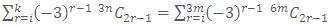Now, (1+x)6m = 6mC0 + 6mC1 x + 6mC2 x2 +...

...+ 6mC6m-1 x6m-1 + 6mC6m x6m          ...... (i)

(1-x)6m = 6mC0 + 6mC1 (-x) + 6mC2 (-x)2 +...

...+ 6mC6m-1 (-x)6m-1 + 6mC6m (-x)6m          ...... (ii)

=> (1+x)6m - (1-x)6m = 2[6mC1x + 6mC3x2 +...+ 6mC6m-1x6m-2]

((1+x)6m - (1-x)6m)/2x = 6mC1 + 6mC3  x2 +......+ 6mC6m-1  x6m - 2

Let x2 = y

=>((1 + √y)6m-(1-√y)6m)/2√y=6mC1+6mC3y  + 6mC3 y+......6mC6m-1 y3m-1

With y = -3, RHS becomes = S.

LHS =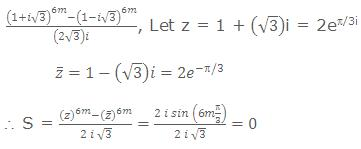(Hence, proved)

Example 11:

If k and n are two positive integers and

Sk = 1k + 2k +.........+ nk

Then show that

m+1C1S1 + m+1C2S2 +......+ m+1CmSm = (1+n)m+1 - (1+n)

Solution:

We have,

(1 + p)m+1 = m+1C0 + m+1C1 p + m+1C2 p2 + m+1Cm+1 pm+1

Putting p = 1, 2, 3, ........., n

=>2m+1 - 1      = m+1C0 + m+1C1(1) + m+1C2(1)2 +...+ m+1Cm(1)m

3m+1 - 2m+1 = m+1C0 + m+1C1(2) + m+1C2(2)2 +...+ m+1Cm(2)m

4m+1 - 3m+1 = m+1C0 + m+1C1(3) + m+1C2(3)2 +...+ m+1Cm(3)m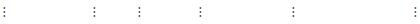(1+n)m+1-nm+1 = m+1C0(n) + m+1C2 Σn + m+1C2 Σ(n)2 +...+ m+1Cm ∑(n)m.

Adding all these terms, we get

(1+n)m+1 -1 = m+1C0(n) + m+1C1S1 + m+1C2S2 +...+ m+1CmSm

=>m+1C1S1 + m+1C2S2 +...+ m+1CmSm = (1+n)m+1 - (1+n)

(Hence, proved)

Example 12:

Given that Sn = 1 + q + q2 +....+ qn

and Pn = 1 + ((q+1)/2) + ((q+1)/2)2 + ....+((q+1)/2)n

show that n+1C1 + n+1C2S1 + n+1C2S2 +...+ n+1Cn+1 Sn = 2n pn.

Solution:

Both Sn and Pn are geometric series

Sn = 1 + q + q2 +......qn = 1-qn-1/1-q                                   ...... (i)

Pn = 1 + ((q+1)/2) + ((q+1)/2)2 +.....+((q+1)/2)n

=(1-((q+1)/2)n+1)/(1-(q+1)/2)=1/2n(2n+1-(q+1)n+1)/(1-q)         ...... (ii)

n+1Cr+1Sr = n+1Cr+1 ((1-qr+1)/(1-q)) =  n+1Cr+1 ((1/(1-q)) - (qr+1/(1-q)))

=  (1/(1-q))n+1Cr+1 -  (1/(1-q)) n+1Cr+1 qr+1

=>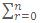n+1Cr+1 Sr=(1/(1-q))n+1Cr+1- (1/(1-q))n+1Cr+1 qr+1

=> ∑n+1Cr+1Sr = 2npn                              (Hence, proved)

Example 13:

Show that if Cr is the coefficient of xr in (1+x)n, thenCr3  = Coefficient of xn yn in the expression of ((1+x)(1+y)(x+y))n

Solution:

(1+x)n = nC0 + nC1 x + nC2 x2 +...+ nCn xn                        ...... (1)

(1+y)n = nC0 + nC1 y + nC2 y2 +...+ nCn yn                         ...... (2)

(x+y)n = nC0 xn + nC1 xn-1 y + nC2 xn-2 +...+ nCn yn          ....... (3)

writing (1+y)n as (y+1)n and expanding it in decreasing powers of y.

(y+1)n = nC0yn + nC1yn-1 + nC2yn-2 +...+ nCn            ....... (4)

multiplying (1), (3) and (4) we get,

(1+x)n (y+1)n (x+y)n

= (nC0 + nC1x + nC2x2 +...+ nCnxn) × (nC0yn + nC1yn-1 +...+ nCn)

× (nC0xn + nC1xn-1y +...+ nCnyn)

Now, coefficient of xnyn in RHS = nC03 + nC13 + nC23 +... nCn3

= coefficient of xnyn in LHS

= coefficient of xnyn in {(1+x)(1+y)(x+y)}n

(Hence, proved)

Example 14:

If (1+x)n = C0 + C1x + C2x2 +...+ Cnxn (nεN)

Then show thatk3 [Ck/Ck-1] = 1/12 (n)(n+1)2(n+2)

Solution:

Cr/Cr-1 = (n!/(n-r)!r!) (((r-1)!(n-r+1)!)/n!) = (((n+1)/r)-1)

r3(Cr/Cr-1) = ((n+1)/r-1) r3 = (n+1)r2 - r3

=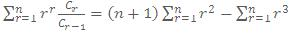(n+1)n(n+1)(2n+1)/6-(n2 (n+1)2)/4

= n(n+1)/12 [4n2 + 6n + 2 - 3n2 - 3n] = n(n+1)(n2+3n+2)/12

n(n+1)(n+1)(n+2)/12=(n(n+1)2 (n+2))/12

(Hence, proved)

Example 15:

If (1+x)n = C0 + C1 x + C2 x2 +...+ Cn xn then show that

(i) ∑0≤i<j≤n  Ci Cj = 22n-1 -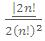(ii) ∑0≤i<j≤n  (Ci + Cj)2 = (n - 1) 2nCn + 22n

(iii) ∑0≤i<j≤n   ∑Ci  Cj = n (22n-1 - 1/2 2nCn)

Solution:

(i)     We have

(C0 + C1 + C2 +...+ Cn)2 = C02 + C12 +...+ Cn2 + 2 ∑0≤i<j≤n   ∑Ci  Cj

we get, (2n)2 = 2nCn + 2 ∑0≤i<j≤n   ∑Ci  Cj

therefore  ∑0≤i<j≤n   ∑Ci  Cj = 22n-1 - (2n)!/2(n!)2

(Hence, proved)

(ii)   ∑0≤i<j≤n   ∑Ci  Cj  n(C02 + C12 + C22 +...+ Cn2) + 2∑0≤i<j≤n   ∑Ci  Cj

= n 2nCn + 2{22n-1 - (2n)!/2(n!)2}               [from part (i)]

= n 2nCn + 22n - 2nCn

= (n-1) 2nCn + 22n

(Hence, proved)

(iii)    Let ∑0≤i<j≤n   ∑Ci  Cj

replace i by (n - i) and j by (n - j), we have

P = ∑0≤i<j≤n   ∑(2n-i0j) Cn-i  Cn-j

=  ∑0≤i<j≤n   ∑(2n-(i+j)) Ci Cj       [·.· nCn = nCn-r]

= 2n ∑0≤i<j≤n  ∑ Ci Cj - P

.·.     ∑0≤i<j≤n   ∑(i+j) Ci Cj = n[22n-1 - (2n)!/2(n!)2]

(Hence, proved)

Register for online classroom programmes targeting IIT JEE 2010 and 2011. You can also participate in the online tests conducted by askIITians and also give answers in AQAD (A Question A Day) to get a number of benefits in the online live classroom courses. Visit askIITians.com to read online Study material for IIT JEE and JEE Main /Advanced (known as AIEEE till 2012) preparation absolutely free.

To read more, Buy study materials of Binomial Theorem comprising study notes, revision notes, video lectures, previous year solved questions etc. Also browse for more study materials on Mathematics here.
```### Course Features

• 731 Video Lectures
• Revision Notes
• Previous Year Papers
• Mind Map
• Study Planner
• NCERT Solutions
• Discussion Forum
• Test paper with Video Solution